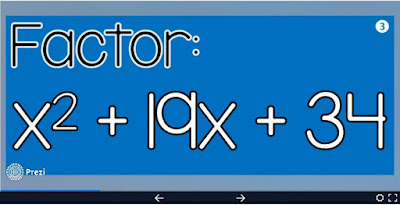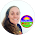## Pages

I love teaching quadratics! It's the unit in Algebra 2 where I see the most student growth and where students seem to be the most proud of what they learn to do. It's just the best unit!

In our classes, we first learn to factor. My students have all seen factoring in Algebra 1 and Geometry, so it's not totally new to them. Still, factoring can feel completely new to some students, so I always start from the very beginning.

In this post are lots of activities and ideas for teaching quadratic functions in algebra class. Since writing it, I've updated with GOOGLE versions of some of the activities.

Over the years, I've made a ton of math cheat sheets to support student learning in my classes. I hand them out at the beginning of a unit and practice referencing it with students so that they can use it on their own. I have found it's one thing to give students references and a whole other to teach them how to use them, so I make sure to set the example. Here is a video and free graphing quadratic functions cheat sheet.

We start without any variables with this simple Let's Factor! powerpoint that gets students thinking the way they'll have to think when factoring, just without the x's.

On our Algebra 2 word wall there are factoring reminder posters that we reference throughout the unit. We also refer to our nonlinear graph references all year. Here is the one for quadratics.

We start by factoring binomials and trinomials where a=1. A couple days in we go through this factoring Prezi as a way for students to self-assess and for me to see who knows (and doesn't yet know) how to factor when a is 1. I give them a simple answer sheet and award classwork participation credit.(zooming factoring Prezi)

Once everyone has a good grasp on factoring trinomials where a=1, we complete this simple factoring activity before moving on to more difficult factoring.

In this activity, students find the lengths and widths of rectangles given quadratic "areas". It's a nice throwback to Geometry and gives factoring a little context. Students can also color their factoring activities.

Another fun way to assess student understanding is with a math pennants. Here is a set of factoring pennants that focuses on trinomials where a=1.

And this set for graphing quadratics

My students always seem to struggle with factoring out greatest common factors. This new factoring trinomials math game called Voyage to the Treasure! includes trinomials with GCFs to factor. After the GCFs are factored out, all A values = 1.

Negative signs are also always tricky, but GCFs are the biggest offender. I made a set of factoring task cards with GCFs for my students that all involve factoring out a GCF as the first step. I find they help remind students to look for GCFs.

After we factor, we move onto the Quadratic Formula. Later when we solve quadratic word problems, my students can choose to solve by factoring or with the Quadratic Formula.

My students have trouble with the structure of the formula and all of the variables, so this Quadratic Formula template really helps. We use it a lot for warm ups. It also works great for quick checks for understanding and as an exit ticket in the last few minutes of class.

I print a stack of the template to have ready to go at the beginning of class. The routine is great for student confidence and knowing that my warm up is ready to go is great for my warm up sanity!

Before templates, I'd always stress about having a meaningful warm up that was long enough or that gave the right sort of practice. To get to know different solution types, I like to slip in this discriminant sorting activity within the first couple days. It gives students more practice evaluating and shows them the pattern for when the Quadratic Formula will yield either 1, 2 or no real solutions.

I find that my students need a lot of practice with the Quadratic Formula and they work through a lot of frustration throughout the process. So to summarize and celebrate their learning we do this fun quadratics chain activity

My students liked the hands-on aspect of getting scissors and cutting the strips. They also really like being able to see their progress right away. There are 12 links and I always give a 100% after 10 are completed correctly.

All of the equations are factorable but my students usually chose to use the Quadratic Formula. When a student completes a problem, I am able to quickly scan the QR code with my QR scanning app.

SOMETHING I LEARNED RECENTLY: Snapchat scans QR codes! Once an answer is correct, it is ready to be made into a chain link. I award 10 points for each correct link and extra credit points if anyone goes beyond 10, which a few students always do.

Here's one of my students holding up his chain in front of one of our math word walls.

Another fun activity to assess student understanding of the Quadratic Formula is this Quadratic Formula pennant activityStudents solve the quadratic on each of the pennants, color and hang them up in the classroom to display their hard work.

My friend Kara at Learning Made Radical and I have started collaborating on these partner scavenger hunts

Each partner gets a clock and their own unique set of problems that yield the same answers. This encourages collaboration as students know their answers should match before moving on to the next problem.

After The Quadratic Formula we move on to quadratic word problems. There are visual supports everywhere in our classroom for this part of our quadratics unit.

This quadratic keywords poster hangs in our room.

I also made this Quadratic Word Problem Keywords Flipbook for student notebooks.

After introducing some of the terminology associated with quadratic word problems, we do this quick quadratic keywords sorting activity to get everyone tuned into how the keywords will present themselves in the problems.

At first, my students complain that there are no numbers on the cards. But then things start to click. They use the keywords poster and think about what each problem is asking them to find.

I find that taking the time to do this sorting activity helps my students feel less intimidated by the word problems once we get to the equations.

I'm not a huge fan of tests, so I use a lot of task cards to assess student understanding. We use 2 sets of task cards with quadratic word problems - quadratic trinomial word problems and another set where the equations are all binomials. These now include a link to a GOOGLE Form version.

Some years I use both sets, other years I use one set or the other. I find that factoring out GCFs can sometimes be hardest for students, so some years we only use this set of quadratic binomial word problem task cardsThese also now include a link to a GOOGLE Form version.

This year my students used the task cards with their graphing calculators. Because the "left bound", "right bound" on the graphing calculator is always tough, this poster hangs in our classroom:

Activities in this post can be found in this Quadratic Functions Activity bundle.quadratic functions activity bundle

I love this unit because of how much my students learn in such a short amount of time. Within a few weeks, they go from having worked primarily with linear equations to finding the amount of time a thrown object is in the air given an initial velocity. It's just so cool!

1.1.2.